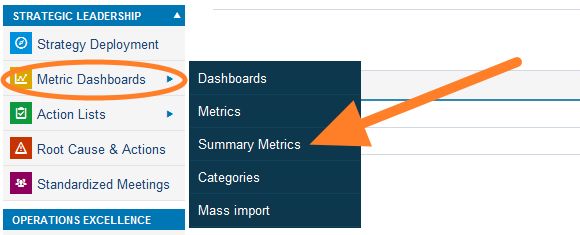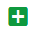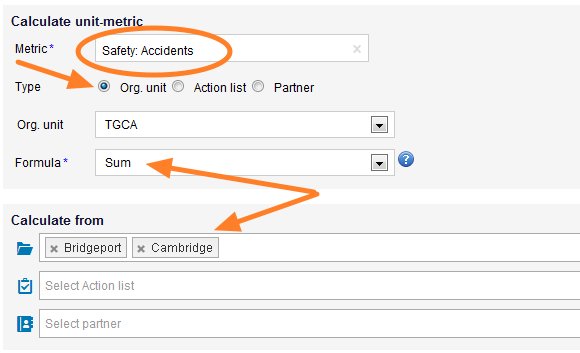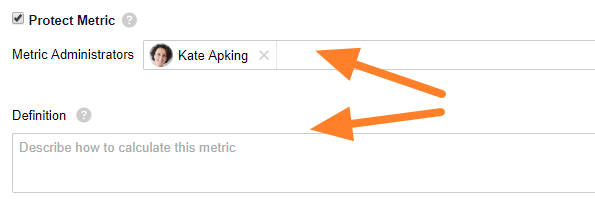Support Center / Metric Dashboards / How to create summary metrics

# How to create summary metrics

You can define how the metric values from selected org. units (e.g. sites) roll up to a higher level org. unit (e.g. region) by using summary metrics.

1. Go to the Metric Dashboards module and select the Summary Metrics in the submenu2. Click the Add icon and choose Create Single Metric3. Select a Metric and a Type (Org. unit, Action list or Partner) for which the value should be calculated
4. Select a Formula for the summary metric (Sum or Average, Minimum or Maximum)
5. Select a particular org. unit(s) or action list(s) or partner(s)  from which the metric should be calculated6. Metric can be also Protected Metric and described in Definition field
7. If you need to roll up a calculated metric (e.g. OTTR, PPM...) you have to create summary metrics for the source values, from which the calculated metric is calculated. Note: for more information on the calculated metrics see calculated-metric-value-counted-from-2-metrics-e-g-ottr-ppm